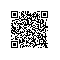# [LintCode] House Robber III 打家劫舍之三

The thief has found himself a new place for his thievery again. There is only one entrance to this area, called the "root." Besides the root, each house has one and only one parent house. After a tour, the smart thief realized that "all houses in this place forms a binary tree". It will automatically contact the police if two directly-linked houses were broken into on the same night.
Determine the maximum amount of money the thief can rob tonight without alerting the police.
Example
3
/ \
2   3
\   \
3   1
Maximum amount of money the thief can rob = 3 + 3 + 1 = 7.
3
/ \
4   5
/ \   \
1   3   1
Maximum amount of money the thief can rob = 4 + 5 = 9.

LeetCode上的原题，请参见我之前的博客House Robber III 。

class Solution {
public:
int houseRobber3(TreeNode* root) {
unordered_map<TreeNode*, int> m;
return helper(root, m);
}
int helper(TreeNode *root, unordered_map<TreeNode*, int> &m) {
if (!root) return 0;
if (m.count(root)) return m[root];
int val = 0;
if (root->left) {
val += helper(root->left->left, m) + helper(root->left->right, m);
}
if (root->right) {
val += helper(root->right->left, m) + helper(root->right->right, m);
}
val = max(val + root->val, helper(root->left, m) + helper(root->right, m));
m[root] = val;
return val;
}
};

class Solution {
public:
int houseRobber3(TreeNode* root) {
vector<int> res = helper(root);
return max(res, res);
}
vector<int> helper(TreeNode *root) {
if (!root) return {0, 0};
vector<int> left = helper(root->left);
vector<int> right = helper(root->right);
vector<int> res{0, 0};
res = max(left, left) + max(right, right);
res = left + right + root->val;
return res;
}
};使用钉钉扫一扫加入圈子
+ 订阅